## Example Questions

← Previous 1

### Example Question #1 : How To Subtract Exponents

If m and n are integers such that m < n < 0 and m2 – n2 = 7, which of the following can be the value of m + n?

I. –5

II. –7

III. –9

I, II and III only

II only

I and II only

I only

II and III only

II only

Explanation:

m and n are both less than zero and thus negative integers, giving us m2 and n2 as perfect squares. The only perfect squares with a difference of 7 is 16 – 9, therefore m = –4 and n = –3.

### Example Question #1 : How To Subtract Exponents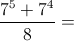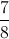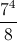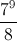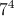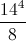Explanation:

To simplify, we can rewrite the numerator using a common exponential base.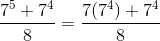Now, we can factor out the numerator.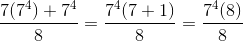The eights cancel to give us our final answer.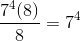### Example Question #1 : How To Subtract Exponents

If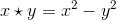, then what is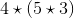?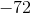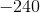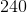Explanation:Follow the order of operations by solving the expression within the parentheses first.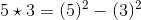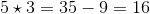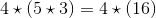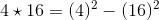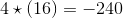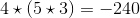### Example Question #1 : How To Subtract Exponents

Simplify the following expression: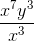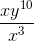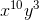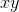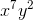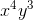Explanation:

The correct answer can be found by subtracting exponents that have the same base. Whenever exponents with the same base are divided, you can subtract the exponent of the denominator from the exponent of the numerator as shown below to obtain the final answer: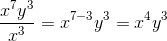You do not do anything with the y exponent because it has no identical bases.

### Example Question #14 : Exponents

Solve: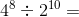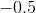Explanation: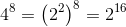Subtract the denominator exponent from the numerator's exponent, since they have the same base.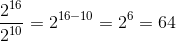### Example Question #2 : How To Subtract Exponents

Simplify: 32 * (423 - 421)

4^4

3^3 * 4^21

3^3 * 4^21 * 5

3^21

3^3 * 4^21 * 5

Explanation:

Begin by noting that the group (423 - 421) has a common factor, namely 421.  You can treat this like any other constant or variable and factor it out.  That would give you: 421(42 - 1). Therefore, we know that:

32 * (423 - 421) = 32 * 421(42 - 1)

Now, 42 - 1 = 16 - 1 = 15 = 5 * 3.  Replace that in the original:

32 * 421(42 - 1) = 32 * 421(3 * 5)

Combining multiples withe same base, you get:

33 * 421 * 5

### Example Question #102 : Exponents

Evaluate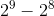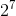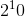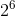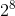Explanation:

When subtracting exponents, we don't multiply the exponents but we try to factor to see if we simplify the subtraction problem. In this case, we can simplify it by factoring. We get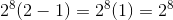.

### Example Question #103 : Exponents

Evaluate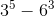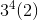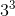Explanation:

Although we have different bases, we know that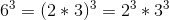. As long as exponents are the same, you are allowed to break the base to its prime numbers. Next, we can factor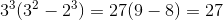### Example Question #2291 : Sat Mathematics

Evaluate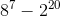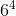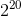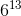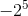Explanation:

Although we have different bases, we know that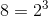. Therefore our expression is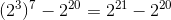. Remember to apply the power rule of exponents. Next, we can factor out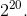We get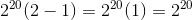.

### Example Question #105 : Exponents

Simplify so that all exponents are positive: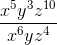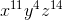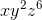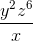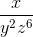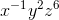Explanation:

When we divide two polynomials with exponents, we subtract their exponents.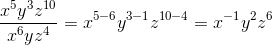Remember that the question asks that all exponents be positive numbers. Therefore: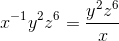← Previous 1

### All SAT Math Resources